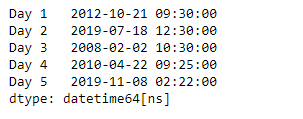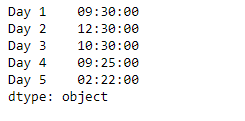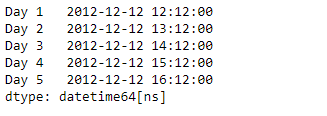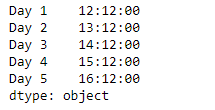Open In App

# Python | Pandas Series.dt.time

`Series.dt` can be used to access the values of the series as datetimelike and return several properties. Pandas` Series.dt.time` attribute return a numpy array of python datetime.time objects.

Syntax: Series.dt.time

Parameter : None

Returns : numpy array

Example #1: Use `Series.dt.time` attribute to return the time property of the underlying data of the given Series object.

 `# importing pandas as pd``import` `pandas as pd`` ` `# Creating the Series``sr ``=` `pd.Series([``'2012-10-21 09:30'``, ``'2019-7-18 12:30'``, ``'2008-02-2 10:30'``,``                ``'2010-4-22 09:25'``, ``'2019-11-8 02:22'``])`` ` `# Creating the index``idx ``=` `[``'Day 1'``, ``'Day 2'``, ``'Day 3'``, ``'Day 4'``, ``'Day 5'``]`` ` `# set the index``sr.index ``=` `idx`` ` `# Convert the underlying data to datetime ``sr ``=` `pd.to_datetime(sr)`` ` `# Print the series``print``(sr)`

Output :Now we will use `Series.dt.time` attribute to return the time property of the underlying data of the given Series object.

 `# return the time``result ``=` `sr.dt.time`` ` `# print the result``print``(result)`

Output :As we can see in the output, the `Series.dt.time` attribute has successfully accessed and returned the time property of the underlying data in the given series object.

Example #2 : Use `Series.dt.time` attribute to return the time property of the underlying data of the given Series object.

 `# importing pandas as pd``import` `pandas as pd`` ` `# Creating the Series``sr ``=` `pd.Series(pd.date_range(``'2012-12-12 12:12'``,``                       ``periods ``=` `5``, freq ``=` `'H'``))`` ` `# Creating the index``idx ``=` `[``'Day 1'``, ``'Day 2'``, ``'Day 3'``, ``'Day 4'``, ``'Day 5'``]`` ` `# set the index``sr.index ``=` `idx`` ` `# Print the series``print``(sr)`

Output :Now we will use `Series.dt.time` attribute to return the time property of the underlying data of the given Series object.

 `# return the time``result ``=` `sr.dt.time`` ` `# print the result``print``(result)`

Output :As we can see in the output, the `Series.dt.time` attribute has successfully accessed and returned the time property of the underlying data in the given series object.